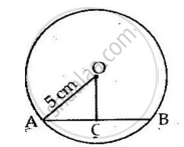Share

A Chord of Length 6 Cm is Drawn in a Circle of Radius 5 Cm. Calculate Its Distance from the Centre of the Circle. - ICSE Class 10 - Mathematics

ConceptChord Properties - the Perpendicular to a Chord from the Center Bisects the Chord (Without Proof)

Question

A chord of length 6 cm is drawn in a circle of radius 5 cm. Calculate its distance from the centre of the circle.

Solution

Let AB be the chord and O be the centre of the circle.
Let OC be the perpendicular drawn from O to AB.We know, that the perpendicular to a chord, from the centre of a circle, bisects the chord.

∴ AC = CB = 3 cm
In ΔOCA,
OA2 = OC2 + AC2 (By Pythagoras theorem)
⟹ OC2 = (5)2 – (3)2 = 16
⟹ OC   = 4 cm

Is there an error in this question or solution?
Solution A Chord of Length 6 Cm is Drawn in a Circle of Radius 5 Cm. Calculate Its Distance from the Centre of the Circle. Concept: Chord Properties - the Perpendicular to a Chord from the Center Bisects the Chord (Without Proof).
S